# Computer generated art with Python¶

## Playing with some simple code¶

#### Roy Keyes¶

21 Sep 2014 - This is a post on my blog.

For a long time I've wanted to do some code generated art. In the past I've messed around with D3 and matplotlib to generate some cool loking graphics, but never looked too deeply into what was possible. I recently stumbled onto this demo of making overlapping plots in matplotlib, and it re-sparked my curiosity about code generated art.

In this post I will show you some very basic techniques and ideas to make some pretty(?), randomly generated art (i.e. plots).

## Scatter plotting is your friend¶

Scatter plotting is an easy way to generate artistic plots, as you can relatively easily control the parameters of every marker on your plot. We'll start with a very basic scatter plot of randomly located points.

In :
# Take care of some environmental stuff
%pylab inline
import matplotlib.pyplot as plt
import numpy as np

figsize(10,10)

Populating the interactive namespace from numpy and matplotlib

In :
bubs = 100

x = np.random.rand(bubs)
y = np.random.rand(bubs)

plt.scatter(x,y)

Out:
<matplotlib.collections.PathCollection at 0x7ff994f90cd0>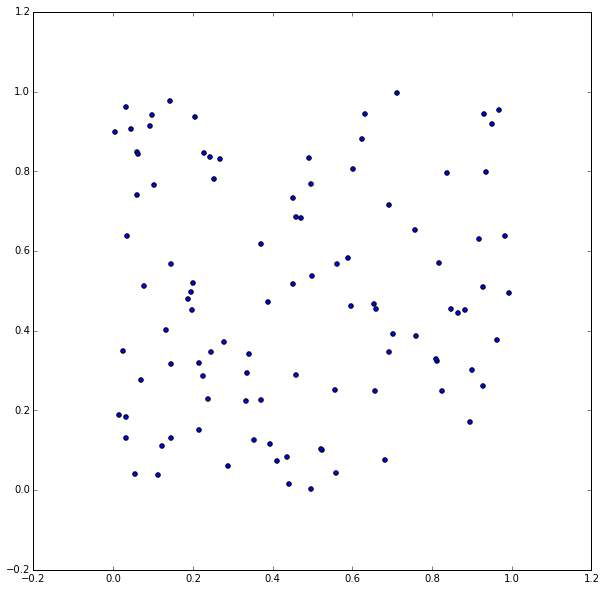Above we've simply plotted random points with the default matplotlib settings. We next add some color. Random of course.

In :
bubs = 100

x = np.random.rand(bubs)
y = np.random.rand(bubs)

colors = plt.cm.jet(np.random.rand(bubs))

plt.scatter(x,y,color=colors)

Out:
<matplotlib.collections.PathCollection at 0x7ff9a898d450>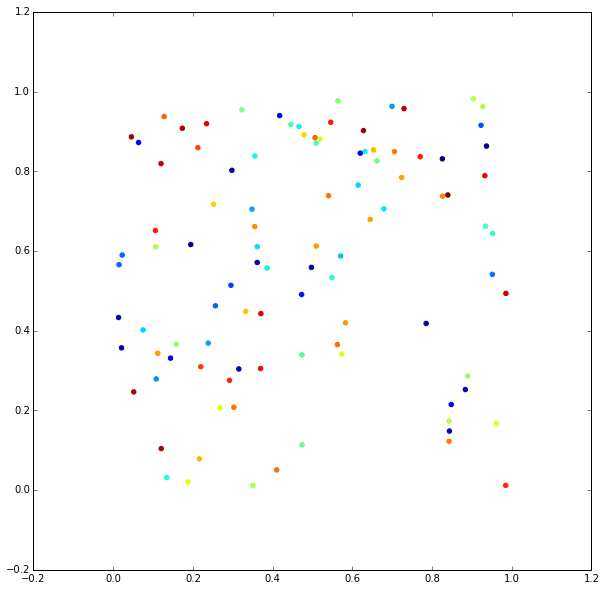Next we randomly vary the marker sizes.

In :
bubs = 100

x = np.random.rand(bubs)
y = np.random.rand(bubs)

colors = plt.cm.jet(np.random.rand(bubs))
sizes = 2*np.pi*(20*np.random.rand(bubs))**2 # Area of markers

plt.scatter(x,y,color=colors,s=sizes)

Out:
<matplotlib.collections.PathCollection at 0x7ff995352b90>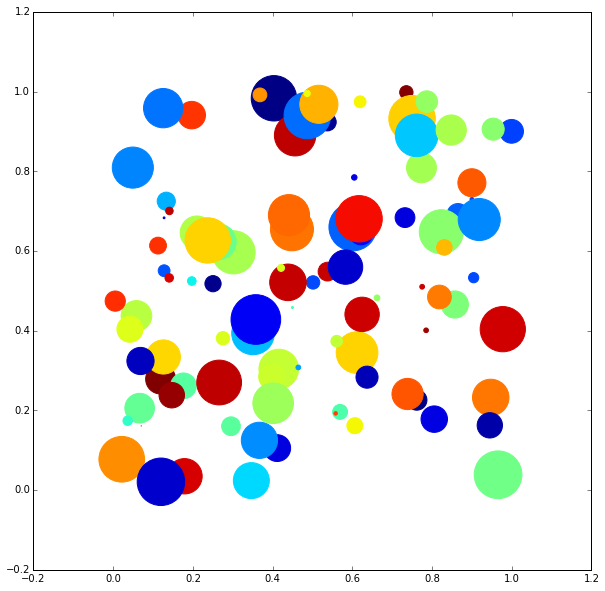Finally, we set the alpha to less than one to get a translucent and more pastel look and remove the edges.

In :
bubs = 100

x = np.random.rand(bubs)
y = np.random.rand(bubs)

colors = plt.cm.jet(np.random.rand(bubs))
sizes = 2*np.pi*(20*np.random.rand(bubs))**2 # Area of markers

plt.scatter(x,y, color=colors, s=sizes, alpha=0.4, lw=0)

Out:
<matplotlib.collections.PathCollection at 0x7ff9a8957e10>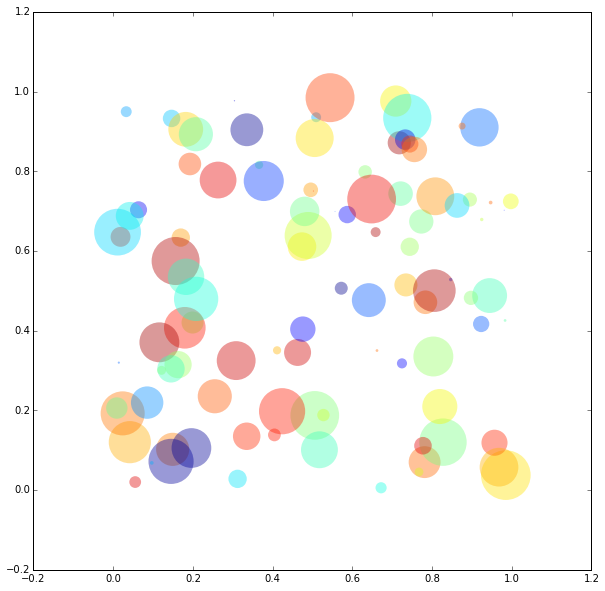#### Bubbles on a path¶

Next I wanted to try generating markers along some trajectory. A simple possibility was to plot markers along a sine curve. In this the position, size, and color are all related to the curve parameters.

Below are a few variations, changing the color generating value and the color map.

In :
bubs = 100
cm = plt.cm.terrain

x = 2*np.pi*np.arange(bubs)/float(bubs)
y = 100*sin(x)
colors = cm(x/x.max())
sizes = np.pi*(8*(x+1))**2

plt.scatter(x, y, color=colors, s=sizes , alpha=0.4, edgecolor='w',lw=3)

Out:
<matplotlib.collections.PathCollection at 0x7ff9aa8c7dd0>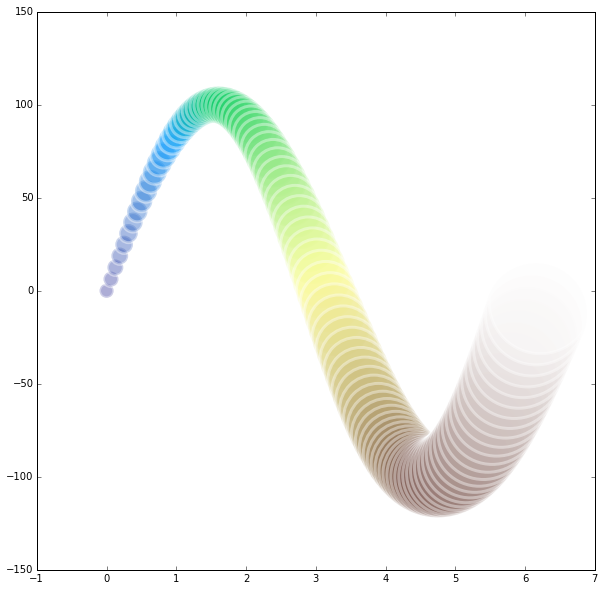In :
bubs = 100
cm = plt.cm.terrain

x = 2*np.pi*np.arange(bubs)/float(bubs)
y = 100*sin(x)
colors = cm(np.random.rand(bubs))
sizes = np.pi*(8*(x+1))**2

plt.scatter(x, y, color=colors, s=sizes , alpha=0.4, edgecolor='w',lw=3)

Out:
<matplotlib.collections.PathCollection at 0x7ff9aa8fe550>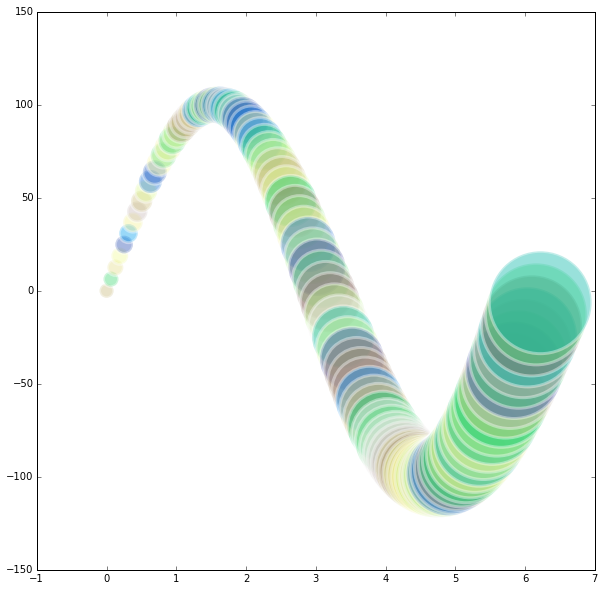In :
bubs = 100
cm = plt.cm.brg

x = 2*np.pi*np.arange(bubs)/float(bubs)
y = 100*sin(x)
colors = cm(np.random.rand(bubs))
sizes = np.pi*(8*(x+1))**2

plt.scatter(x, y, color=colors, s=sizes , alpha=0.4, edgecolor='w',lw=3)

Out:
<matplotlib.collections.PathCollection at 0x7ff9aaa1c510>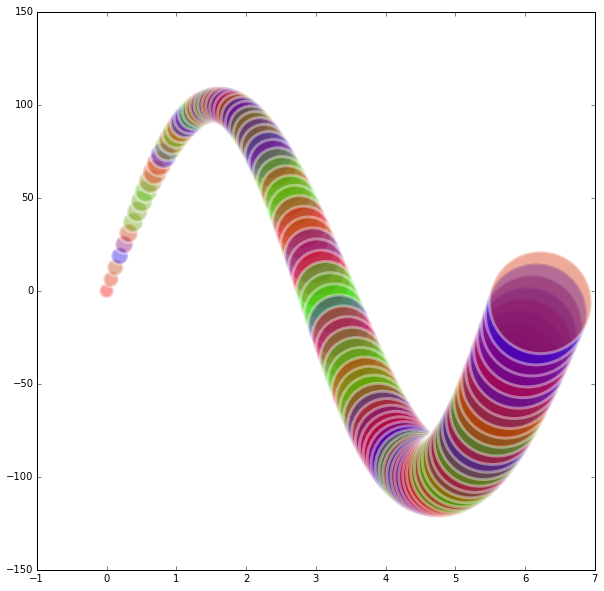### Bubble necklace¶

Next I decided to attempt a more "necklace" look, varying the sizes randomly.

In :
bubs = 100
cm = plt.cm.terrain

x = 2*np.pi*np.arange(bubs)/float(bubs)
y = 100*sin(x)
colors = cm(np.random.rand(bubs))
sizes = np.pi*(10*np.random.rand(bubs))**2

plt.scatter(x, y, color=colors, s=sizes , alpha=0.5, edgecolor='w',lw=3)

Out:
<matplotlib.collections.PathCollection at 0x5dc2890>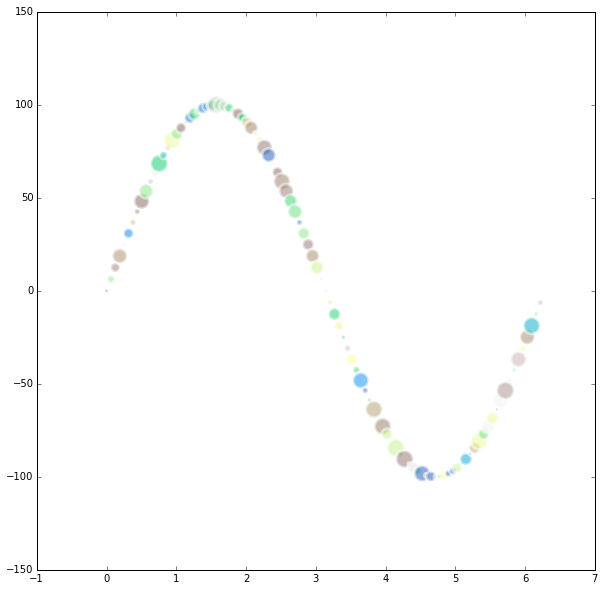### Spiral of bubbles¶

For the last art plot I decided to try to make a spiral of bubbles. This is very similar to the previous, but following a spiral path, thanks to a little math.

In :
# Spiral
num_points = 200
p = linspace(0,10*np.pi,num_points)

x = 10*p*sin(p)
y = 10*p*cos(p)

p1 = np.log(1+p/10)
colors = cm(np.append(p1[:99],p1[99::-1]))
sizes = 5*p*2*np.pi*(0.5+2*np.random.rand(num_points))**2

plt.scatter(x,y, color=colors, s=sizes, alpha=0.5, edgecolor='k', linewidth=0)

Out:
<matplotlib.collections.PathCollection at 0x7ff997886990>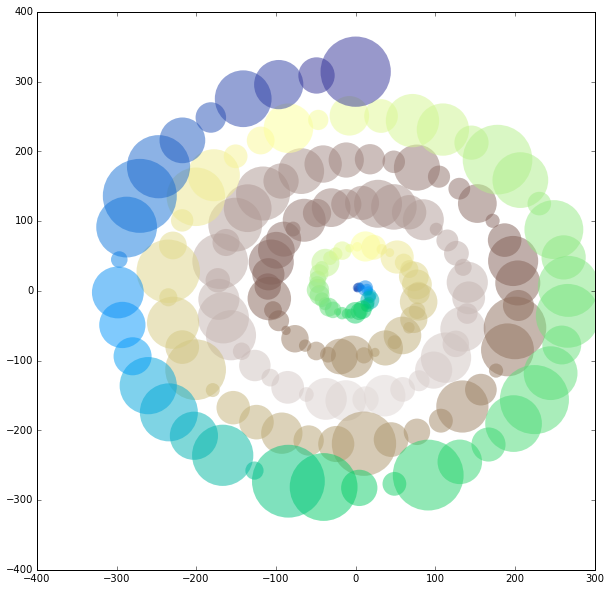### art.generate_masterpiece()¶

I hope you enjoyed these very basic forays into code generated art!